• 主要利用高博书中第5讲相机模型的知识解决相机畸变校正和双目点云生成的问题
1.有相机内参的情况下，对畸变图像进行处理
去畸变公式：image_undistorted(u,v)=image_raw(u_distorted,v_distorted)
1.直接获取原图的(u,v)，就是去畸变后的像素坐标------------>去畸变后的归一化坐标（x,y）
2.由去畸变后的归一化坐标（x,y）--------------->计算得到去畸变前归一化坐标（x_distorted,y_distorted）
3.由去畸变前归一化坐标（x_distorted,y_distorted）------------>计算得到去畸变前(u_distorted,v_distorted)
这里直接获取原图的(u,v)是去畸变后的像素坐标有点难理解，可以看作去畸变后的尺寸和原始的图像一样，只是拿他的尺寸用，不用(u,v)对应的值，最后将原图与之对应的去畸变前(u_distorted,v_distorted)的像素值给image_undistorted(u,v)
//author:jiangcheng

#include <opencv2/opencv.hpp>
#include <string>

using namespace std;

string image_file = "./test.png";   // 请确保路径正确

int main(int argc, char **argv) {

// 本程序需要你自己实现去畸变部分的代码。尽管我们可以调用OpenCV的去畸变，但自己实现一遍有助于理解。
// 畸变参数
double k1 = -0.28340811, k2 = 0.07395907, p1 = 0.00019359, p2 = 1.76187114e-05;
// 内参
double fx = 458.654, fy = 457.296, cx = 367.215, cy = 248.375;

cv::Mat image = cv::imread(image_file,0);   // 图像是灰度图，CV_8UC1
int rows = image.rows, cols = image.cols;
cv::Mat image_undistort = cv::Mat(rows, cols, CV_8UC1);   // 去畸变以后的图

// 计算去畸变后图像的内容
for (int v = 0; v < rows; v++)
for (int u = 0; u < cols; u++) {

double u_distorted = 0, v_distorted = 0;
// TODO 按照公式，计算点(u,v)对应到畸变图像中的坐标(u_distorted, v_distorted) (~6 lines)
// start your code here
// 1.当前u,v为去畸变之后的像素坐标(考虑好的图片的u,v的值对应原始图片中的u_dis,v_dis)
//求出去畸变后的归一化坐标
double x_undistorted=(u-cx)/fx;
double x=x_undistorted;
double y_undistorted=(v-cy)/fy;
double y=y_undistorted;
double r=sqrt(x*x+y*y);
//计算去畸变前的归一化坐标
double x_distorted=x*(1+k1*r*r+k2*pow(r,4))+2*p1*x*y+p2*(r*r+2*x*x);
double y_distorted=y*(1+k1*r*r+k2*r*r*r*r)+2*p2*x*y+p1*(r*r+2*y*y);
//计算去畸变前的像素坐标
u_distorted=x_distorted*fx+cx;
v_distorted=y_distorted*fy+cy;
// end your code here

// 赋值 (最近邻插值)
if (u_distorted >= 0 && v_distorted >= 0 && u_distorted < cols && v_distorted < rows) {
image_undistort.at<uchar>(v, u) = image.at<uchar>((int) v_distorted, (int) u_distorted);
} else {
image_undistort.at<uchar>(v, u) = 0;
}
}

// 画图去畸变后图像
cv::imshow("image undistorted", image_undistort);
cv::imwrite("undistorted_test_img.png",image_undistort);
cv::waitKey();

return 0;
}


原始图片：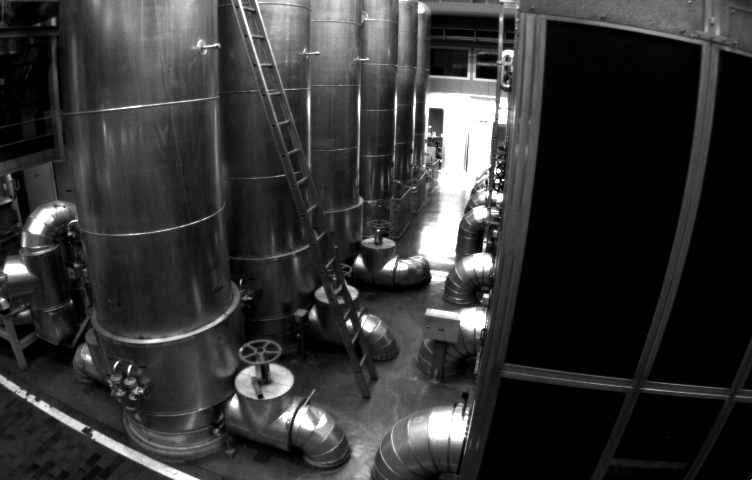去畸变后的图片：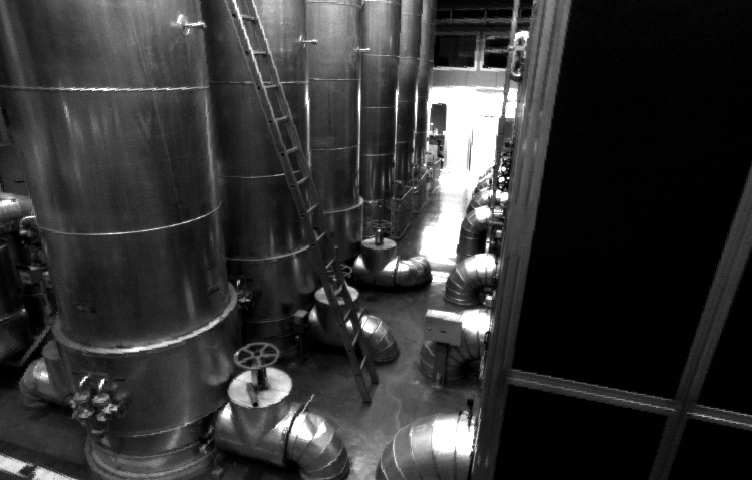2.双目生成点云
利用左目的图像信息和视差图信息就能生成点云图像
利用以下公式计算出像素对应的三维点云：
double z = (fx * d * 1000) / disp;//计算中是以毫米为单位的；
point = z ;
point = (u - cx) * point / fx;
point = (v - cy) * point / fy;
#include <opencv2/opencv.hpp>
#include <string>
#include <Eigen/Core>
#include <pangolin/pangolin.h>
#include <unistd.h>

using namespace std;
using namespace Eigen;

// 文件路径，如果不对，请调整
string left_file = "./left.png";
string right_file = "./right.png";
string disparity_file = "./disparity.png";

// 在panglin中画图，已写好，无需调整
void showPointCloud(const vector<Vector4d, Eigen::aligned_allocator<Vector4d>> &pointcloud);

int main(int argc, char **argv) {

// 内参
double fx = 718.856, fy = 718.856, cx = 607.1928, cy = 185.2157;
// 间距
double d = 0.573;

// 读取图像
cv::Mat left = cv::imread(left_file, 0);
cv::Mat right = cv::imread(right_file, 0);
cv::Mat disparity = cv::imread(disparity_file, 0); // disparty 为CV_8U,单位为像素

// 生成点云
vector<Vector4d, Eigen::aligned_allocator<Vector4d>> pointcloud;

// TODO 根据双目模型计算点云
// 如果你的机器慢，请把后面的v++和u++改成v+=2, u+=2
for (int v = 0; v < left.rows; v++)
for (int u = 0; u < left.cols; u++) {

Vector4d point(0, 0, 0, left.at<uchar>(v, u) / 255.0); // 前三维为xyz,第四维为颜色

// start your code here (~6 lines)
// 根据双目模型计算 point 的位置

unsigned int disp = disparity.ptr<unsigned short>(v)[u];
if(disp==0){
cout << "disp=0" << endl;
continue; }
double z = (fx * d * 1000) / disp;//计算中是以毫米为单位的；
point = z ;
point = (u - cx) * point / fx;
point = (v - cy) * point / fy;
// cout << "point = [" << point << ", "
// << point << ", "   << point <<", " << point <<  "]" << endl;
pointcloud.push_back(point);
// end your code here
}

// 画出点云
showPointCloud(pointcloud);
return 0;
}

void showPointCloud(const vector<Vector4d, Eigen::aligned_allocator<Vector4d>> &pointcloud) {

if (pointcloud.empty()) {
cerr << "Point cloud is empty!" << endl;
return;
}

pangolin::CreateWindowAndBind("Point Cloud Viewer", 1024, 768);
glEnable(GL_DEPTH_TEST);
glEnable(GL_BLEND);
glBlendFunc(GL_SRC_ALPHA, GL_ONE_MINUS_SRC_ALPHA);

pangolin::OpenGlRenderState s_cam(
pangolin::ProjectionMatrix(1024, 768, 500, 500, 512, 389, 0.1, 1000),
pangolin::ModelViewLookAt(0, -0.1, -1.8, 0, 0, 0, 0.0, -1.0, 0.0)
);

pangolin::View &d_cam = pangolin::CreateDisplay()
.SetBounds(0.0, 1.0, pangolin::Attach::Pix(175), 1.0, -1024.0f / 768.0f)
.SetHandler(new pangolin::Handler3D(s_cam));

while (pangolin::ShouldQuit() == false) {
glClear(GL_COLOR_BUFFER_BIT | GL_DEPTH_BUFFER_BIT);

d_cam.Activate(s_cam);
glClearColor(1.0f, 1.0f, 1.0f, 1.0f);

glPointSize(2);
glBegin(GL_POINTS);
for (auto &p: pointcloud) {
glColor3f(p, p, p);
glVertex3d(p, p, p);
}
glEnd();
pangolin::FinishFrame();
usleep(5000);   // sleep 5 ms
}
return;
}


点云显示：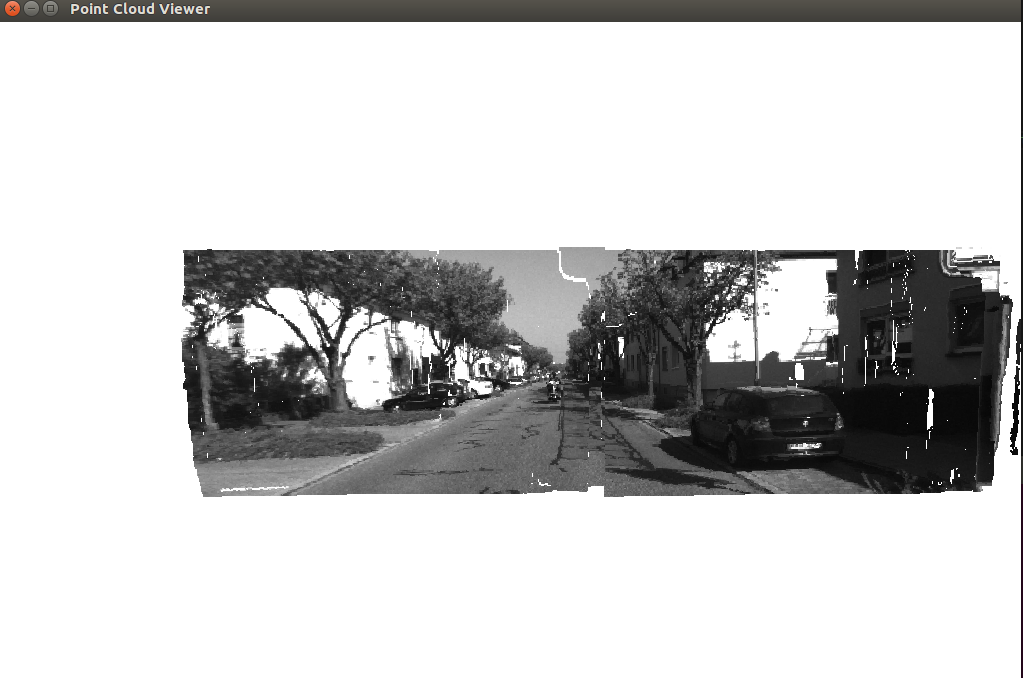展开全文• 对此，提出了一种基于双目点云重建单目点云的方法，系统无需增加其他操作过程，单次扫描就能同时获得双目点云和精度较高的左右单目点云。在对飞机模型的测量中，利用该方法填补了双目测量在机翼附近出现的数据丢失，...三维重建
• 视觉SLAM-深蓝学院-第五讲-双目生成点云 前言 双目生成点云这道题，很多人，包括我做的时候深度都有问题。如下图。这是别人博客上的一张结果，我做的时候也是这样，仔细观察可以发现，在这里深度图的宽是原图的...
视觉SLAM-深蓝学院-第五讲-双目生成点云
前言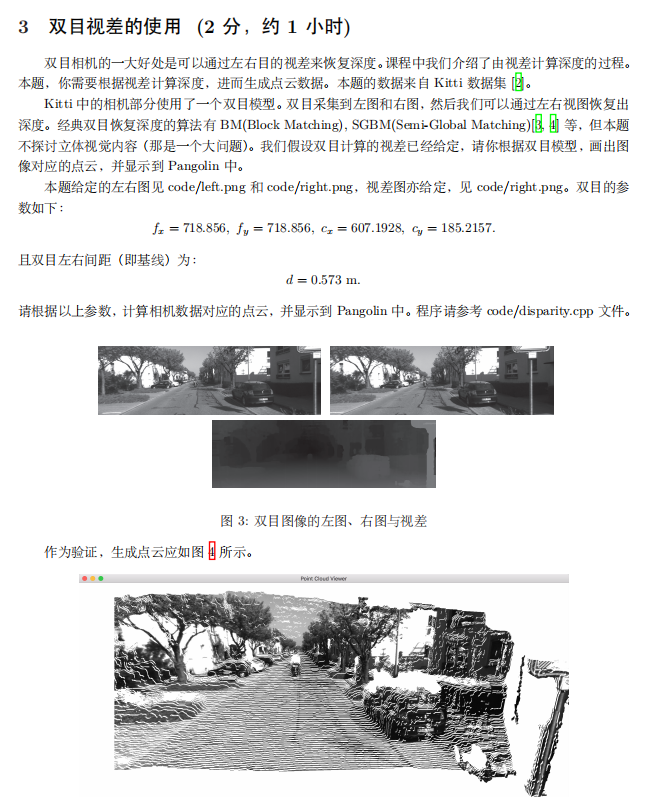双目生成点云这道题，很多人，包括我做的时候深度都有问题。如下图。这是别人博客上的一张结果，我做的时候也是这样，仔细观察可以发现，在这里深度图的宽是原图的一半，深度图读了两次，图中间可以看到一个电线杆的形状，右边又可以看到一个电线杆。但是原深度图的长宽是没有问题的。这涉及到png的交错存储问题，可以理解成隔行扫描，故问题就很明显了。解决方法有很多种：

转换png格式，改为无交错
修改程序，如下（有其他改法）

    int main(int argc, char **argv) {
// 内参
double fx = 718.856, fy = 718.856, cx = 607.1928, cy = 185.2157;
// 间距
double b = 0.573;
// 读取图像
cv::Mat left = cv::imread(left_file, 0);
cv::Mat right = cv::imread(right_file, 0);
cv::Mat disparity =
cv::imread(disparity_file,0);  // disparty 为CV_8U,单位为像素
// 生成点云
vector<Vector4d, Eigen::aligned_allocator<Vector4d>> pointcloud;
// TODO 根据双目模型计算点云
// 如果你的机器慢，请把后面的v++和u++改成v+=2, u+=2
for (int v = 0; v < left.rows; v++){
for (int u = 0; u < left.cols; u++) {
Vector4d point(
0, 0, 0,
left.at<uchar>(v, u) / 255.0);  // 前三维为xyz,第四维为颜色
// start your code here (~6 lines)
// 根据双目模型计算 point 的位置
unsigned short d = disparity.at<unsigned short>(v, u/2);
point = 200 * log(65536.0 / double(d) + 1);
point = (u - cx) * point / fx;
point = (v - cy) * point / fy;
pointcloud.push_back(point);
// end your code here
}
}
// 画出点云
showPointCloud(pointcloud);
return 0;
}

结果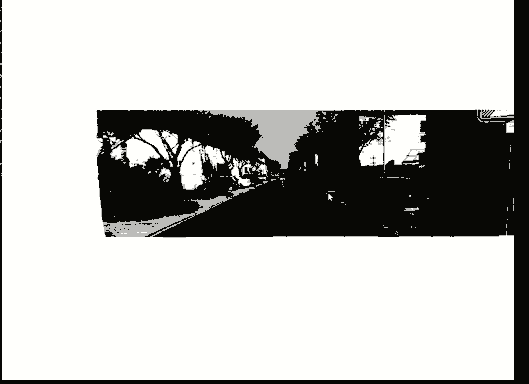展开全文视觉SLAM
• 双目视觉，点云原理： http://www.elecfans.com/d/863829.html 单目视觉测距代码： https://www.cnblogs.com/fpzs/p/9513932.html
双目视觉，点云原理：
http://www.elecfans.com/d/863829.html
单目视觉测距代码：
https://www.cnblogs.com/fpzs/p/9513932.html


展开全文• 使用 Matlab 生成双目视差及点云图像 准备工作 采集棋盘格图像 使用 Matlab Stereo Camera Calibrator 进行双目相机标定 编写 matlab 脚本，生成视差图，点云图（TODO） 使用 Matlab 生成双目视差及点云图像...
目录使用 Matlab 生成双目视差及点云图像（TODO）准备工作采集棋盘格图像使用 Matlab *Stereo Camera Calibrator* 进行双目相机标定编写 matlab 脚本，生成视差图，点云图matlab 脚本运行结果说明参考
使用 Matlab 生成双目视差及点云图像（TODO）
最近需要验证一下双相机的成像效果，一开始使用了opencv + python/cpp 代码采集、标定并生成视差图，效果不是很理想，所以决定先用 matlab 标定相机，生成图像点云，查看效果，如果不错的话，以此为基准优化 opencv 代码。
准备工作
首先需要下载 Matlab（废话，哈哈哈），请支持正版，还需要在其中安装名为 Stereo Camera Calibrator 的立体相机标定程序包。图标是这个样子的（截图好麻烦，算了）。当然，对于 Matlab 菜鸟来说，资料手册什么的必不可少（摸着书本过河比无脑乱撞的要好）。所以下面几个资料还是推荐一下了（官网英文板的）：

Computer Vision ToolBox 官方网页，跟着教程说明走，不懂就 csdn + StackOverflow，对了，如果可以的话，最好是在这个页面下载 Computer Vision ToolBox 的 PDF 文档（包含 Computer Vision Toolbox Getting Started Guide，Computer Vision Toolbox User’s Guide（以后简称手册） 以及另外两个我认为不重要的），不过需要在 Matlab 注册邮箱
同上（😃）

好了，开始搞事！下面简单记录下实现步骤，免得以后忘了。
采集棋盘格图像
很无聊的一项工作，但是还是有些要注意的地方。在 Computer Vision ToolBox User’s Guide 中对单目以及双目相机的标定图案、步骤有很详细很规范的说明，以前看 OpenCV 文档的时候的一些模棱两可的操作在这里面都表达的很清楚。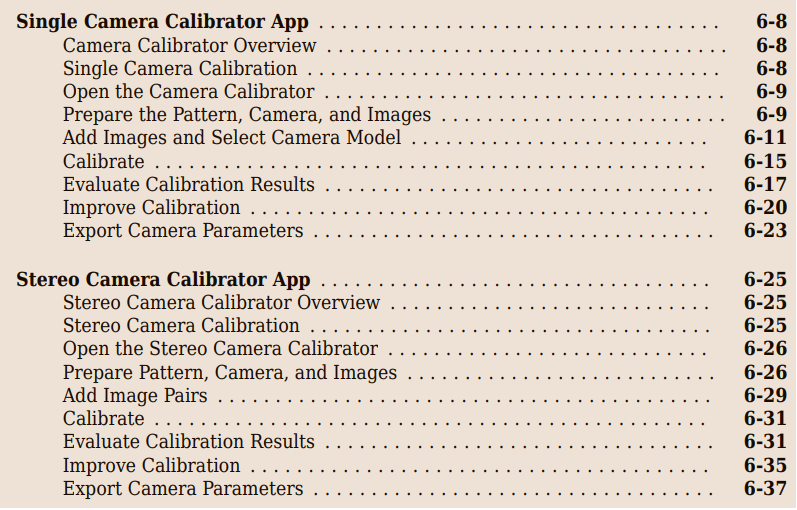具体的操作步骤参考手册把，列出几个以前犯错误的地方，以示提醒。

标定板一边的棋盘格数与另一边的必须奇偶互斥，如果是 12x9 的棋盘格，那么此棋盘格的角点数为 11x8 共 88 个（每条边角点数=此边棋盘格数-1）。
相机在采集图像前需要使用分辨率板进行对焦校准。分辨率板如图。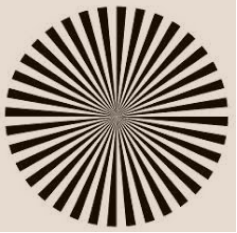不要使用自动对焦功能。
每改变一次焦距，就要重新标定相机。
用于标定图像数目在10-20张之间，所以尽可能地多采集一些图像（对于普通的非工业相机来说），因为很多情况下超过50%的图像最后是要被筛选掉的。
图像最好保存为无损格式.png，.bmp。
标定板的位置尽量放置在双目相机测量物体的距离之间。比如想在1.5米处测量物体，就在大约1.5米处放置标定板。
采集标定板图像时，可以将标定板多放置几个旋转角度（我没怎么旋转标定板），但是，标定板与相机光轴的倾角不要大于45度。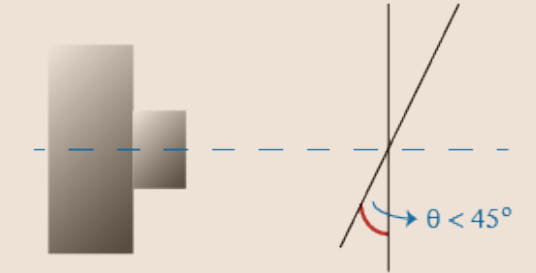标定板尽量要大一些，这样可以使它在标定时占据图像大部分区域；也就是说，如果需要在很近的距离标定相机（近距离成像），就选相对较小的标定板，反正大的标定板。
标定板在一对双目图像中必须各个棋盘格可见。

采集的标定图像示例（标定板有些小，只显示了左相机图像）
注意，需要将左右图集分别放在同级的两个不同的目录下，比如
*
|-left
|-left_*.png
|-right
|-right_*.png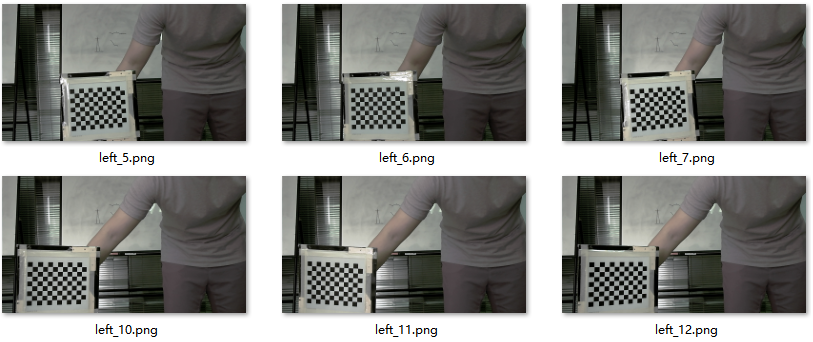使用 Matlab Stereo Camera Calibrator 进行双目相机标定
接下来就是操作matlab的标定包了。

首先打开matlab，在 App 中选择 Stereo Camera Calibrator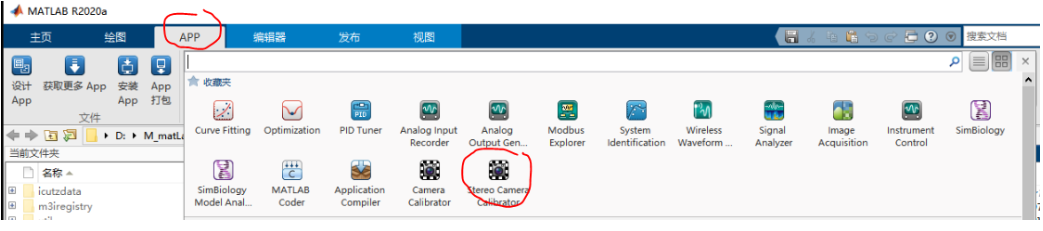打开后选择 Add Image（1号），在弹出的对话框中分别给定左右图像集的搜索路径，然后指定棋盘格的大小（单位：mm）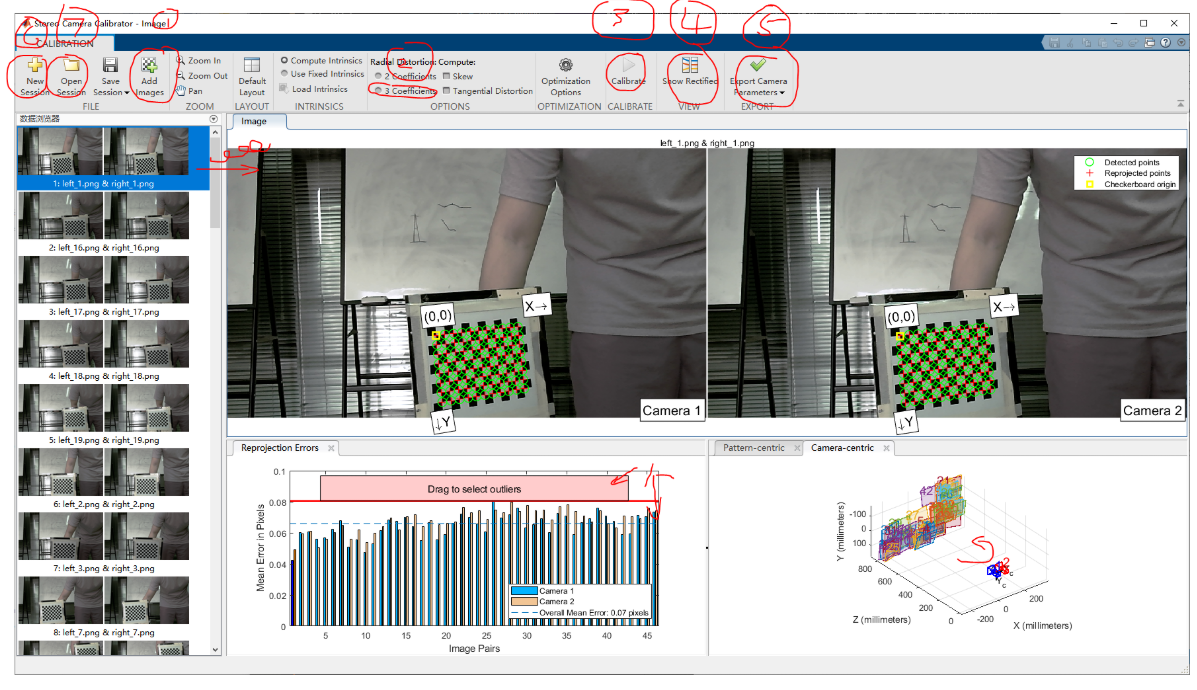可以选择 3 Coefficient（2号）（出处：手册page204，如下图） 使标定更准确。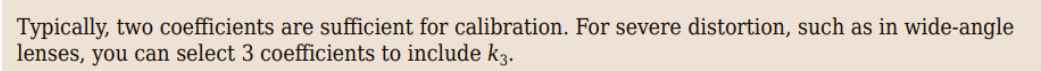点击 Calibrate（3号）对双目图像进行标定，标定后可以计算出 reprojection error (再次投影误差) 并以条形图显示，可以通过上下拖拽红色区域批量删除误差值过高的图像对，也可以通过 ctrl+鼠标左键多选要删除的不符合要求的图像，被选择的图像在左侧显示栏中以蓝色背景显示，可以直接右键其中的任何一组图像对进行批量删除。我的删图标准，如下；通常情况下，Overall Mean Error（平均误差达） 在 0.06 左右就可以了。

图像对中任何一个或一对图像误差值过大的
接着是图像对中左右图误差值只差过大的
reprojection error (再次投影误差) 如图（放大处理），还没有查是如何计算出来的以及代表着什么（TODO）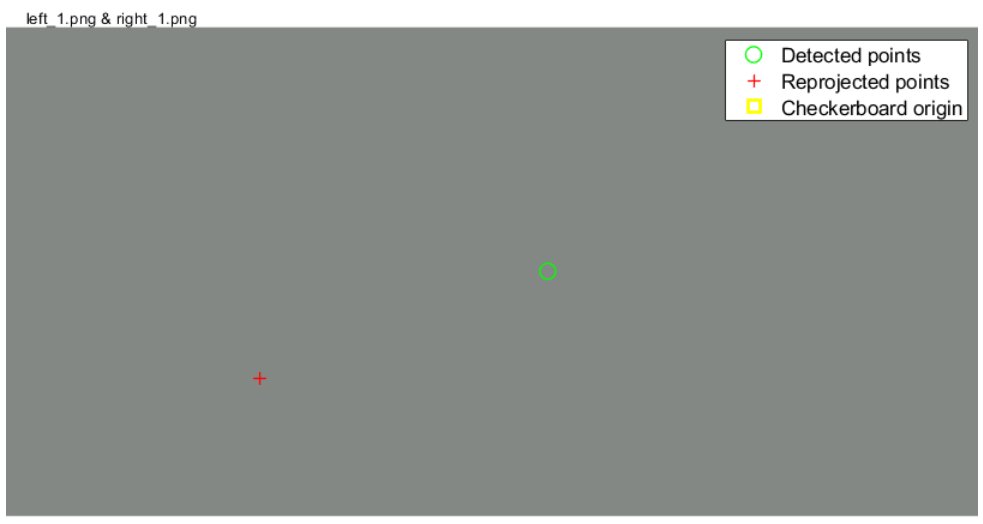点击 Show Rectified，对图像对进行y轴（水平对齐），输出图如下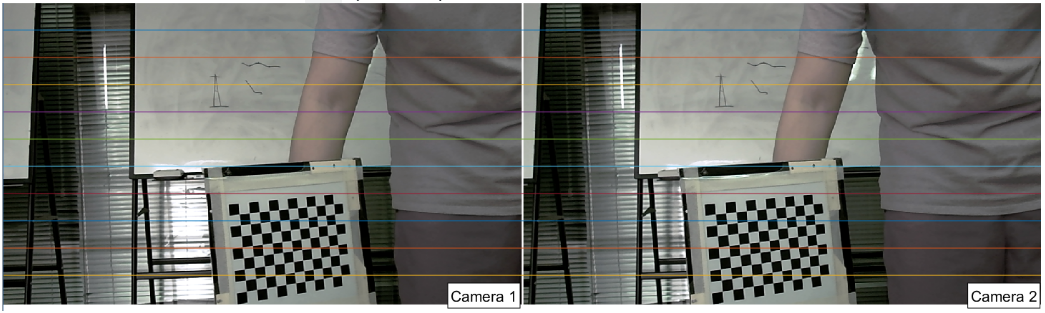接着点击 Export Camera Parameters（5号），会出现两个选项的下拉列表，Export Parameters to workspace 将标定的内外参数矩阵，投影误差等导出到 Matlab 主程序界面的工作区，Generate Matlab script 生成标定运算的脚本。
最后记得保存这次的 session 标定会话，也可以创建或导出保存的会话（6，7号）

编写 matlab 脚本，生成视差图，点云图
matlab 脚本
run("D:\M_matLab2020a\workSpace\522d_0605\run_522d_0605.m");

% 读取左右彩色图像
I1_C3 = imread("D:\M_matLab2020a\workSpace\522d_0605\object_left\left_0.png");
I2_C3 = imread("D:\M_matLab2020a\workSpace\522d_0605\object_right\right_0.png");

% 矫正左右彩色图像，利用导入的立体相机参数
[J1_C3, J2_C3] = rectifyStereoImages(I1_C3, I2_C3, stereoParams);

% 利用矫正后的左右图生成立体图像
Anaglyph = stereoAnaglyph(J1_C3, J2_C3);
figure; imshow(Anaglyph);

% 使用 imtool 确定视差范围值（max=93.5），这里取 128，（具体操作见说明）
figure; imtool(Anaglyph);
disparityRange = [0, 128];

% 利用矫正后的灰度图生成视差图
J1_C1 = rgb2gray(J1_C3);
J2_C1 = rgb2gray(J2_C3);

% Minimum value of uniqueness = 20
disparityMap_t20 = disparitySGM(J1_C1, J2_C1, 'DisparityRange', disparityRange, 'UniquenessThreshold', 20);
figure; imshow(disparityMap_t20, disparityRange); title('disp t20'); colormap jet; colorbar;

% Minimum value of uniqueness = 15
disparityMap_t15 = disparitySGM(J1_C1, J2_C1, 'DisparityRange', disparityRange, 'UniquenessThreshold', 15);
figure; imshow(disparityMap_t15, disparityRange); title('disp t15'); colormap jet; colorbar;

% Minimum value of uniqueness = 10
disparityMap_t10 = disparitySGM(J1_C1, J2_C1, 'DisparityRange', disparityRange, 'UniquenessThreshold', 10);
figure; imshow(disparityMap_t10, disparityRange); title('disp t10'); colormap jet; colorbar;

% Minimum value of uniqueness = 5
disparityMap_t5 = disparitySGM(J1_C1, J2_C1, 'DisparityRange', disparityRange, 'UniquenessThreshold', 5);
figure; imshow(disparityMap_t5, disparityRange); title('disp t5'); colormap jet; colorbar;

% Minimum value of uniqueness = 0
disparityMap_t0 = disparitySGM(J1_C1, J2_C1, 'DisparityRange', disparityRange, 'UniquenessThreshold', 0);
figure; imshow(disparityMap_t0, disparityRange); title('disp t0'); colormap jet; colorbar;

% 利用视差图重建 3D 图
points3D = reconstructScene(disparityMap_t10, stereoParams);

% 将距离单位由 mm -> m
points3D = points3D ./ 1000;

% 存储 3D 图的点云数据
ptCloud = pointCloud(points3D, 'Color', J1_C3);

% 使用 pcplayer 观察点云图
player3D = pcplayer([-1, 1], [-1, 1], [0, 2], 'VerticalAxis', 'Y', 'VerticalAxisDir', 'Up');
view(player3D, ptCloud);

运行结果
原始图（投影了散斑激光）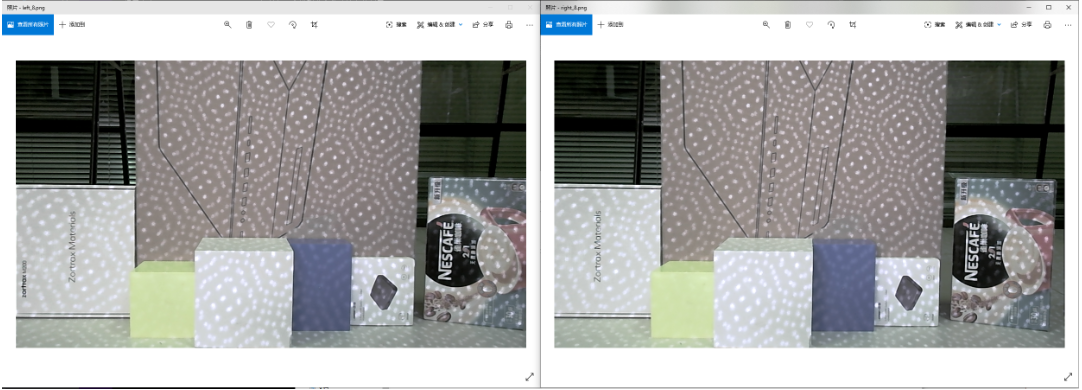视差图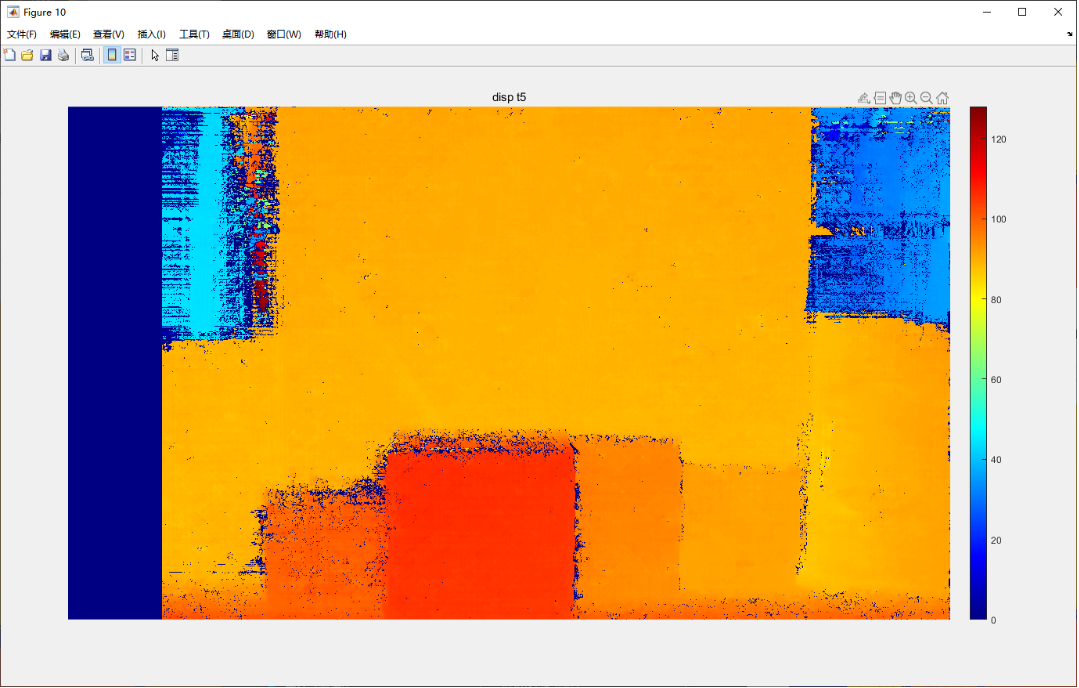点云图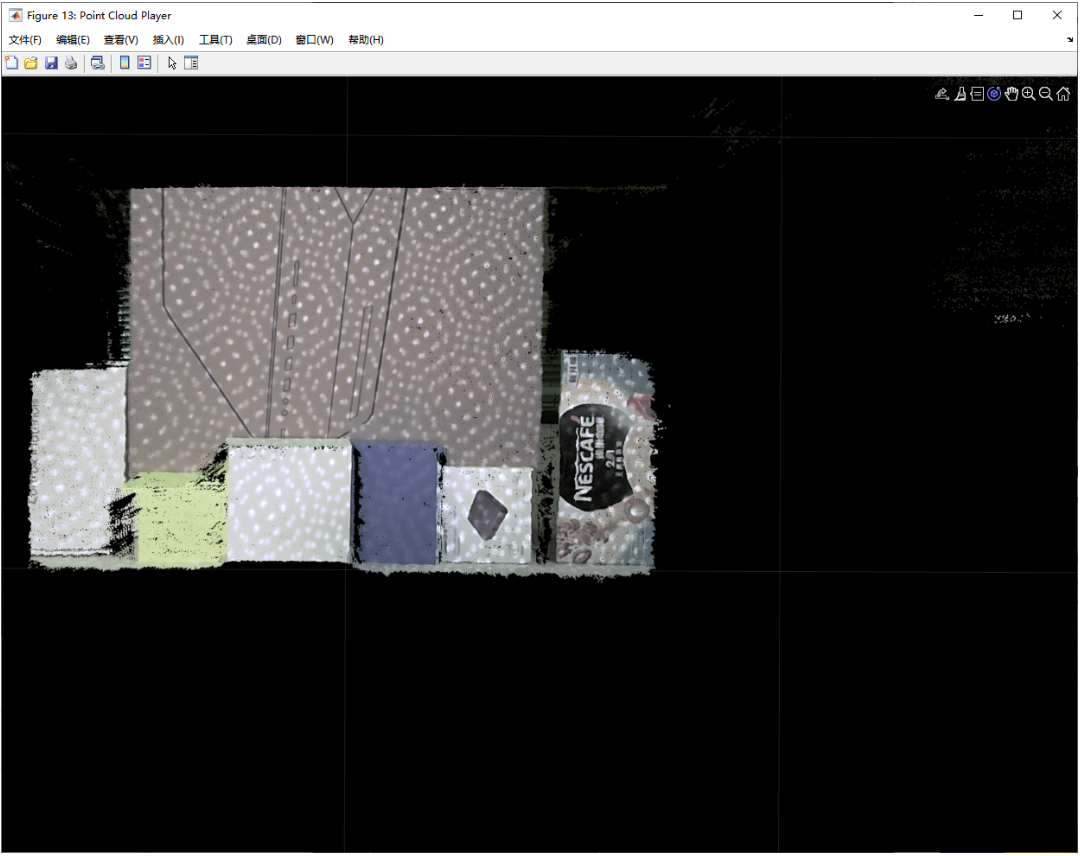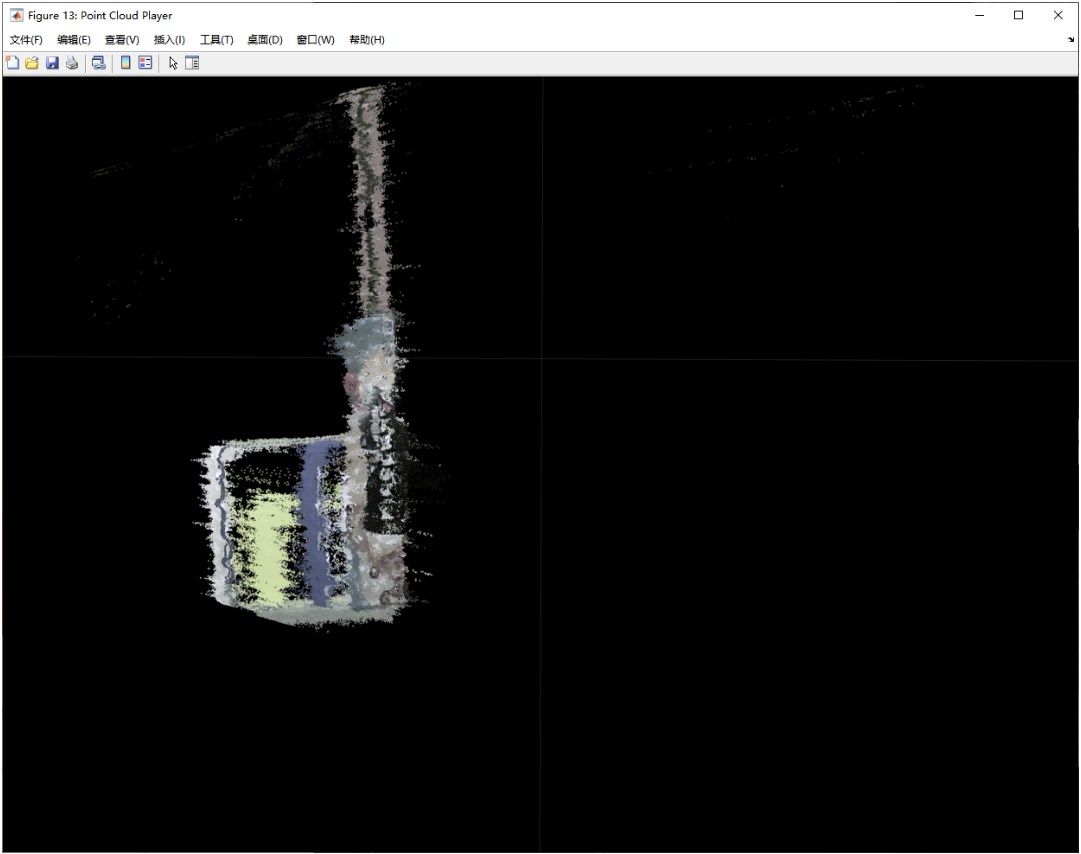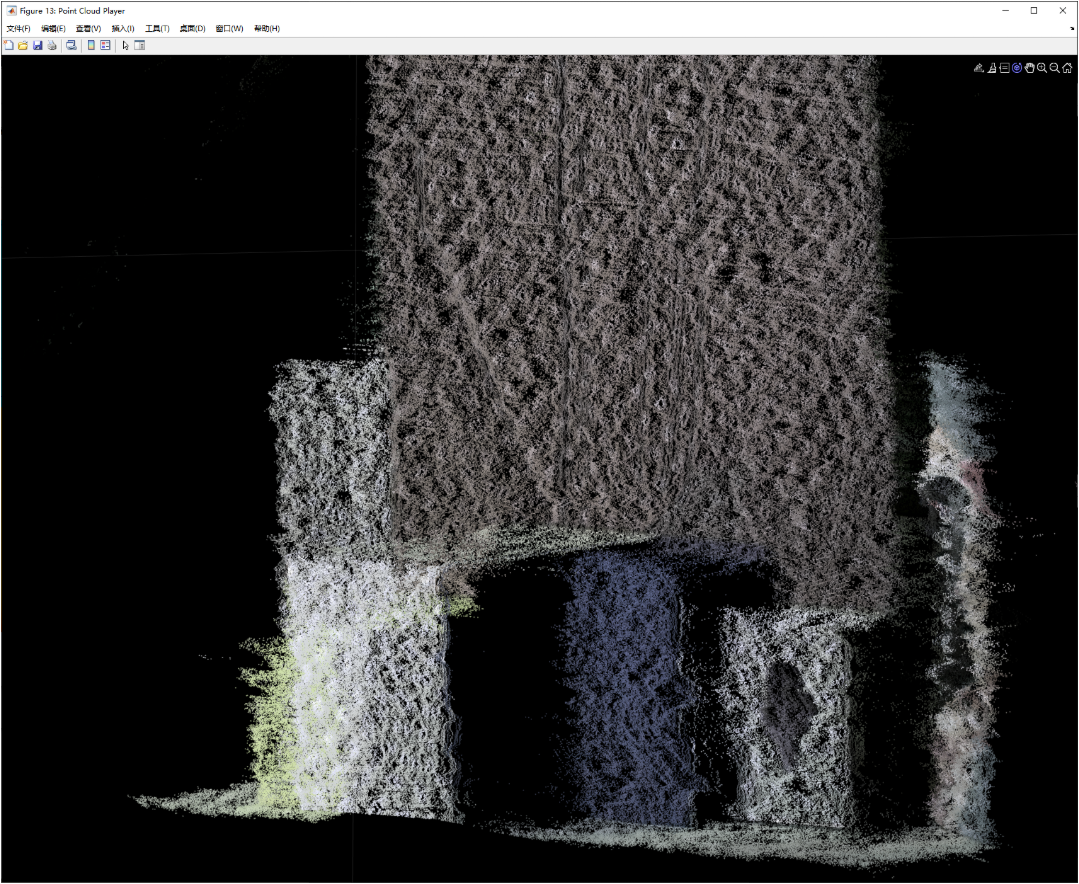说明
确定视差范围
使用 imtool 工具测量近中远三个物体的视差距离如图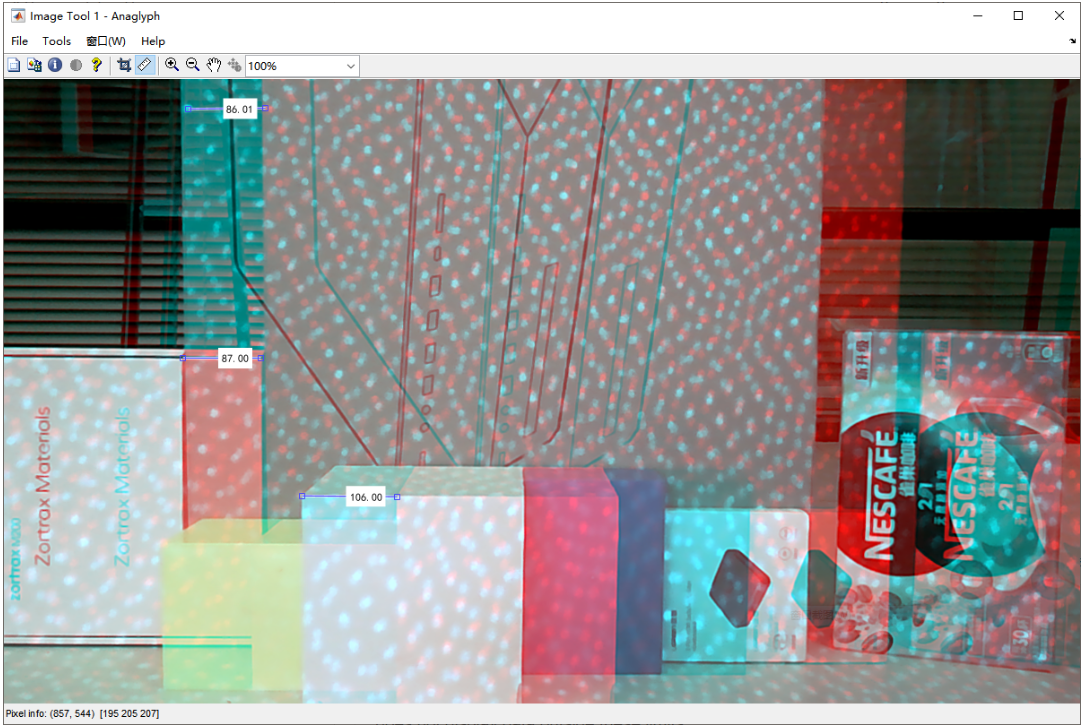最大视差为 106，则视差范围设为 [0, 128]
参考

matlab 教程
Reconstruct 3-D scene from disparity map
Compute disparity map through semi-global matchin
Depth Estimation From Stereo Video
stereoParameters


展开全文• 利用单相机中已获得的可靠相位数据,提出一种双目视觉相位匹配中的视差孔洞数据插补方法。该方法在测量系统的标定...对人脸面具和葫芦模型进行双目测量和孔洞插补实验,结果证明该方法可以很好地补全遮挡区域缺失的点云
• // TODO 根据双目模型计算点云 // 如果你的机器慢，请把后面的v++和u++改成v+=2, u+=2 for (int v = 0; v ; v++) for (int u = 0; u ; u++) { Vector4d point(0, 0, 0, left.at(v, u) / 255.0); // 前三维为...
• ## 双目相机计算稠密深度点云（一）

千次阅读 多人点赞 2020-08-28 14:57:59
这篇博客主要记录如何使用双目相机计算点云数据 双目计算深度点云的ROS包有两个： stereo_image_proc 和 LIBELAS（: Library for Efficient Large-scale Stereo Matching）两个库，其中LIBELAS提供了一个ROS环境下的...双目立体匹配原理
• OpenCV 双目视差图生成点云变成这样 双目视差图生成点云变成这样，大家知道怎么回事吗 校正图像与视差图opencv
• 笔者提出了一种基于双目视觉稀疏点云重建的输电线路弧垂测量方法,通过获取电力走廊影像对,采用数字图像处理技术对输电线路进行定位与提取,使用标定参数采样核线影像对,并利用核线约束解算其稀疏点云,最后通过悬链线...
• // 根据双目模型计算 point 的三维空间位置 double x = (u - cx) / fx; double y = (v - cy) / fy; double depth = fx * b / (disparity.at(v, u)); point = x * depth; point = y * depth; point = depth...视觉slam
• 双目相机计算稠密深度点云 在这里我接着上一篇的文章实现利用真实的相机计算3D点云，实验中我们使用的是ZED的相机。ZED 双目立体匹配
• 众所周知，获取场景深度点云信息是计算机视觉系统中的重要作用之一，通过深度点云信息，计算机视觉系统可获取场景内各点距双目相机的位置，提供图像测距、深度图像分割、三维目标识别等功能，进而可对真实场景进行三...
• 在上一篇博客ORB-SLAM2 在线构建稠密点云（室内RGBD篇）中介绍了如何通过深度相机和ORB_SLAM2实现稠密点云建图，并转换为octomap在ROS中显示，那么这篇文章将使用双目相机实现室外的稠密点云构建。与深度相机不同，......